# Mean State

Period Mean (original grids) [W/m2]
Model Period Mean (intersection) [W/m2]
Model Period Mean (complement) [W/m2]
Benchmark Period Mean (intersection) [W/m2]
Benchmark Period Mean (complement) [W/m2]
Bias [W/m2]
RMSE [W/m2]
Phase Shift [months]
Bias Score 
RMSE Score 
Seasonal Cycle Score 
Spatial Distribution Score 
Interannual Variability Score 
Overall Score 
Benchmark [-] 457.
CRUNCEPv7 [-] 464. 464. 0.00 456. 465. 8.32 17.6 1.41 0.42 0.38 0.80 0.88 0.62 0.58
GSWP3v1 [-] 463. 463. 0.00 456. 465. 7.83 16.4 1.44 0.46 0.38 0.80 0.98 0.61 0.60
WATCH [-] 455. 454. 0.00 454. 466. 1.45 12.5 2.20 0.49 0.32 0.68 1.0 0.67 0.58
Period Mean (original grids) [W/m2]
Model Period Mean (intersection) [W/m2]
Model Period Mean (complement) [W/m2]
Benchmark Period Mean (intersection) [W/m2]
Benchmark Period Mean (complement) [W/m2]
Bias [W/m2]
RMSE [W/m2]
Phase Shift [months]
Bias Score 
RMSE Score 
Seasonal Cycle Score 
Spatial Distribution Score 
Interannual Variability Score 
Overall Score 
Benchmark [-] 466.
CRUNCEPv7 [-] 487. 487. 0.00 467. 458. 20.4 26.5 0.743 0.41 0.48 0.93 1.0 0.64 0.66
GSWP3v1 [-] 484. 484. 0.00 467. 458. 17.2 23.5 0.637 0.46 0.50 0.94 0.95 0.62 0.66
WATCH [-] 465. 465. 0.00 467. 459. -2.24 19.2 1.03 0.64 0.44 0.89 1.0 0.73 0.69
Period Mean (original grids) [W/m2]
Model Period Mean (intersection) [W/m2]
Model Period Mean (complement) [W/m2]
Benchmark Period Mean (intersection) [W/m2]
Benchmark Period Mean (complement) [W/m2]
Bias [W/m2]
RMSE [W/m2]
Phase Shift [months]
Bias Score 
RMSE Score 
Seasonal Cycle Score 
Spatial Distribution Score 
Interannual Variability Score 
Overall Score 
Benchmark [-] 443.
CRUNCEPv7 [-] 450. 450. 0.00 443. 446. 7.78 19.3 1.24 0.56 0.47 0.85 0.99 0.63 0.66
GSWP3v1 [-] 452. 452. 0.00 443. 446. 9.13 18.8 1.38 0.57 0.46 0.81 1.0 0.60 0.65
WATCH [-] 442. 442. 0.00 441. 445. 2.13 18.6 1.59 0.63 0.41 0.79 1.0 0.68 0.65
Period Mean (original grids) [W/m2]
Model Period Mean (intersection) [W/m2]
Model Period Mean (complement) [W/m2]
Benchmark Period Mean (intersection) [W/m2]
Benchmark Period Mean (complement) [W/m2]
Bias [W/m2]
RMSE [W/m2]
Phase Shift [months]
Bias Score 
RMSE Score 
Seasonal Cycle Score 
Spatial Distribution Score 
Interannual Variability Score 
Overall Score 
Benchmark [-] 279.
CRUNCEPv7 [-] 268. 268. 0.00 278. 296. -8.32 17.5 0.132 0.86 0.80 0.99 1.0 0.71 0.86
GSWP3v1 [-] 277. 278. 0.00 278. 296. 2.37 15.4 0.127 0.90 0.81 0.99 1.0 0.74 0.88
WATCH [-] 267. 267. 0.00 279. 294. -9.59 17.3 0.132 0.85 0.77 0.99 1.0 0.73 0.85
Period Mean (original grids) [W/m2]
Model Period Mean (intersection) [W/m2]
Model Period Mean (complement) [W/m2]
Benchmark Period Mean (intersection) [W/m2]
Benchmark Period Mean (complement) [W/m2]
Bias [W/m2]
RMSE [W/m2]
Phase Shift [months]
Bias Score 
RMSE Score 
Seasonal Cycle Score 
Spatial Distribution Score 
Interannual Variability Score 
Overall Score 
Benchmark [-] 457.
CRUNCEPv7 [-] 457. 457. 0.00 454. 467. 3.95 14.6 1.88 0.30 0.37 0.71 0.92 0.54 0.54
GSWP3v1 [-] 459. 459. 0.00 454. 467. 5.54 14.6 1.55 0.29 0.38 0.77 0.65 0.58 0.51
WATCH [-] 456. 456. 0.00 455. 468. 2.65 13.8 2.37 0.16 0.35 0.63 0.75 0.56 0.46
Period Mean (original grids) [W/m2]
Model Period Mean (intersection) [W/m2]
Model Period Mean (complement) [W/m2]
Benchmark Period Mean (intersection) [W/m2]
Benchmark Period Mean (complement) [W/m2]
Bias [W/m2]
RMSE [W/m2]
Phase Shift [months]
Bias Score 
RMSE Score 
Seasonal Cycle Score 
Spatial Distribution Score 
Interannual Variability Score 
Overall Score 
Benchmark [-] 312.
CRUNCEPv7 [-] 311. 310. 0.00 311. 334. -0.679 9.44 0.0515 0.94 0.89 1.0 0.99 0.86 0.93
GSWP3v1 [-] 317. 317. 0.00 311. 334. 5.51 11.6 0.0521 0.91 0.88 1.0 1.0 0.86 0.92
WATCH [-] 309. 309. 0.00 315. 334. -6.11 9.78 0.292 0.91 0.85 0.98 0.99 0.87 0.91
Period Mean (original grids) [W/m2]
Model Period Mean (intersection) [W/m2]
Model Period Mean (complement) [W/m2]
Benchmark Period Mean (intersection) [W/m2]
Benchmark Period Mean (complement) [W/m2]
Bias [W/m2]
RMSE [W/m2]
Phase Shift [months]
Bias Score 
RMSE Score 
Seasonal Cycle Score 
Spatial Distribution Score 
Interannual Variability Score 
Overall Score 
Benchmark [-] 364.
CRUNCEPv7 [-] 363. 362. 0.00 363. 399. 0.922 14.0 0.225 0.86 0.82 0.98 1.0 0.80 0.88
GSWP3v1 [-] 366. 365. 0.00 363. 399. 4.07 14.2 0.164 0.86 0.82 0.99 1.0 0.76 0.87
WATCH [-] 358. 358. 0.00 368. 403. -8.30 15.0 0.285 0.80 0.78 0.98 1.0 0.80 0.86
Period Mean (original grids) [W/m2]
Model Period Mean (intersection) [W/m2]
Model Period Mean (complement) [W/m2]
Benchmark Period Mean (intersection) [W/m2]
Benchmark Period Mean (complement) [W/m2]
Bias [W/m2]
RMSE [W/m2]
Phase Shift [months]
Bias Score 
RMSE Score 
Seasonal Cycle Score 
Spatial Distribution Score 
Interannual Variability Score 
Overall Score 
Benchmark [-] 448.
CRUNCEPv7 [-] 459. 459. 0.00 446. 467. 14.4 21.7 0.742 0.51 0.56 0.92 1.0 0.67 0.71
GSWP3v1 [-] 453. 454. 0.00 446. 467. 9.50 18.9 0.825 0.60 0.56 0.91 0.98 0.63 0.71
WATCH [-] 449. 450. 0.00 449. 468. 3.35 20.2 1.20 0.57 0.49 0.86 0.99 0.63 0.67
Period Mean (original grids) [W/m2]
Model Period Mean (intersection) [W/m2]
Model Period Mean (complement) [W/m2]
Benchmark Period Mean (intersection) [W/m2]
Benchmark Period Mean (complement) [W/m2]
Bias [W/m2]
RMSE [W/m2]
Phase Shift [months]
Bias Score 
RMSE Score 
Seasonal Cycle Score 
Spatial Distribution Score 
Interannual Variability Score 
Overall Score 
Benchmark [-] 281.
CRUNCEPv7 [-] 275. 276. 0.00 281. 288. -4.81 13.7 0.101 0.91 0.81 0.99 0.99 0.78 0.88
GSWP3v1 [-] 284. 284. 0.00 281. 288. 4.17 13.2 0.0840 0.91 0.83 0.99 1.0 0.77 0.89
WATCH [-] 277. 277. 0.00 282. 286. -4.49 14.0 0.299 0.91 0.78 0.97 1.0 0.82 0.88
Period Mean (original grids) [W/m2]
Model Period Mean (intersection) [W/m2]
Model Period Mean (complement) [W/m2]
Benchmark Period Mean (intersection) [W/m2]
Benchmark Period Mean (complement) [W/m2]
Bias [W/m2]
RMSE [W/m2]
Phase Shift [months]
Bias Score 
RMSE Score 
Seasonal Cycle Score 
Spatial Distribution Score 
Interannual Variability Score 
Overall Score 
Benchmark [-] 441.
CRUNCEPv7 [-] 449. 449. 0.00 443. 426. 3.69 13.5 0.384 0.78 0.70 0.97 0.98 0.80 0.82
GSWP3v1 [-] 449. 449. 0.00 443. 426. 3.62 13.2 0.381 0.80 0.69 0.97 0.99 0.74 0.81
WATCH [-] 443. 443. 0.00 438. 425. 2.20 15.9 0.625 0.80 0.64 0.94 0.99 0.71 0.79
Period Mean (original grids) [W/m2]
Model Period Mean (intersection) [W/m2]
Model Period Mean (complement) [W/m2]
Benchmark Period Mean (intersection) [W/m2]
Benchmark Period Mean (complement) [W/m2]
Bias [W/m2]
RMSE [W/m2]
Phase Shift [months]
Bias Score 
RMSE Score 
Seasonal Cycle Score 
Spatial Distribution Score 
Interannual Variability Score 
Overall Score 
Benchmark [-] 368.
CRUNCEPv7 [-] 362. 363. 0.00 366. 389. -0.870 12.0 0.447 0.85 0.77 0.97 1.0 0.80 0.86
GSWP3v1 [-] 365. 365. 0.00 366. 389. 1.41 11.8 0.429 0.86 0.77 0.97 1.0 0.78 0.86
WATCH [-] 360. 360. 0.00 370. 391. -7.02 12.4 0.210 0.79 0.74 0.99 0.99 0.77 0.84
Period Mean (original grids) [W/m2]
Model Period Mean (intersection) [W/m2]
Model Period Mean (complement) [W/m2]
Benchmark Period Mean (intersection) [W/m2]
Benchmark Period Mean (complement) [W/m2]
Bias [W/m2]
RMSE [W/m2]
Phase Shift [months]
Bias Score 
RMSE Score 
Seasonal Cycle Score 
Spatial Distribution Score 
Interannual Variability Score 
Overall Score 
Benchmark [-] 399.
CRUNCEPv7 [-] 378. 378. 0.00 376. 409. 3.26 16.3 0.561 0.71 0.67 0.94 1.0 0.69 0.78
GSWP3v1 [-] 381. 381. 0.00 376. 409. 6.29 16.1 0.539 0.72 0.67 0.94 1.0 0.69 0.78
WATCH [-] 374. 374. 0.00 378. 409. -2.45 15.9 0.785 0.71 0.64 0.91 1.0 0.74 0.77
Period Mean (original grids) [W/m2]
Model Period Mean (intersection) [W/m2]
Model Period Mean (complement) [W/m2]
Benchmark Period Mean (intersection) [W/m2]
Benchmark Period Mean (complement) [W/m2]
Bias [W/m2]
RMSE [W/m2]
Phase Shift [months]
Bias Score 
RMSE Score 
Seasonal Cycle Score 
Spatial Distribution Score 
Interannual Variability Score 
Overall Score 
Benchmark [-] 450.
CRUNCEPv7 [-] 457. 457. 0.00 450. 446. 6.78 16.8 0.377 0.81 0.76 0.97 0.99 0.71 0.83
GSWP3v1 [-] 452. 452. 0.00 450. 446. 2.24 15.1 0.358 0.84 0.76 0.97 1.0 0.72 0.84
WATCH [-] 437. 437. 0.00 453. 450. -15.3 20.3 0.581 0.70 0.71 0.94 1.0 0.75 0.80
Period Mean (original grids) [W/m2]
Model Period Mean (intersection) [W/m2]
Model Period Mean (complement) [W/m2]
Benchmark Period Mean (intersection) [W/m2]
Benchmark Period Mean (complement) [W/m2]
Bias [W/m2]
RMSE [W/m2]
Phase Shift [months]
Bias Score 
RMSE Score 
Seasonal Cycle Score 
Spatial Distribution Score 
Interannual Variability Score 
Overall Score 
Benchmark [-] 281.
CRUNCEPv7 [-] 278. 278. 0.00 281. 293. -3.57 15.7 0.202 0.90 0.79 0.98 0.99 0.71 0.86
GSWP3v1 [-] 287. 286. 0.00 281. 293. 6.06 16.8 0.168 0.89 0.79 0.99 1.0 0.76 0.87
WATCH [-] 283. 283. 0.00 284. 293. -1.54 15.6 0.339 0.91 0.77 0.97 1.0 0.78 0.87
Period Mean (original grids) [W/m2]
Model Period Mean (intersection) [W/m2]
Model Period Mean (complement) [W/m2]
Benchmark Period Mean (intersection) [W/m2]
Benchmark Period Mean (complement) [W/m2]
Bias [W/m2]
RMSE [W/m2]
Phase Shift [months]
Bias Score 
RMSE Score 
Seasonal Cycle Score 
Spatial Distribution Score 
Interannual Variability Score 
Overall Score 
Benchmark [-] 442.
CRUNCEPv7 [-] 446. 446. 0.00 439. 459. 11.3 19.0 0.815 0.51 0.55 0.90 1.0 0.69 0.70
GSWP3v1 [-] 447. 447. 0.00 439. 459. 12.0 18.6 0.885 0.51 0.56 0.89 0.95 0.67 0.69
WATCH [-] 445. 445. 0.00 441. 459. 7.00 18.3 1.21 0.53 0.53 0.84 0.99 0.72 0.69
Period Mean (original grids) [W/m2]
Model Period Mean (intersection) [W/m2]
Model Period Mean (complement) [W/m2]
Benchmark Period Mean (intersection) [W/m2]
Benchmark Period Mean (complement) [W/m2]
Bias [W/m2]
RMSE [W/m2]
Phase Shift [months]
Bias Score 
RMSE Score 
Seasonal Cycle Score 
Spatial Distribution Score 
Interannual Variability Score 
Overall Score 
Benchmark [-] 386.
CRUNCEPv7 [-] 388. 388. 0.00 384. 429. 6.07 11.6 0.309 0.86 0.85 0.98 0.99 0.87 0.90
GSWP3v1 [-] 391. 391. 0.00 384. 429. 8.89 13.0 0.112 0.82 0.86 0.99 0.99 0.85 0.90
WATCH [-] 384. 384. 0.00 387. 429. -1.29 10.5 0.525 0.88 0.82 0.96 0.99 0.87 0.89
Period Mean (original grids) [W/m2]
Model Period Mean (intersection) [W/m2]
Model Period Mean (complement) [W/m2]
Benchmark Period Mean (intersection) [W/m2]
Benchmark Period Mean (complement) [W/m2]
Bias [W/m2]
RMSE [W/m2]
Phase Shift [months]
Bias Score 
RMSE Score 
Seasonal Cycle Score 
Spatial Distribution Score 
Interannual Variability Score 
Overall Score 
Benchmark [-] 324.
CRUNCEPv7 [-] 323. 323. 0.00 323. 347. 0.502 10.9 0.135 0.91 0.84 0.99 0.99 0.78 0.89
GSWP3v1 [-] 328. 328. 0.00 323. 347. 5.87 13.2 0.104 0.88 0.83 0.99 0.97 0.78 0.88
WATCH [-] 325. 325. 0.00 328. 349. -1.80 11.5 0.281 0.90 0.81 0.98 0.99 0.80 0.88
Period Mean (original grids) [W/m2]
Model Period Mean (intersection) [W/m2]
Model Period Mean (complement) [W/m2]
Benchmark Period Mean (intersection) [W/m2]
Benchmark Period Mean (complement) [W/m2]
Bias [W/m2]
RMSE [W/m2]
Phase Shift [months]
Bias Score 
RMSE Score 
Seasonal Cycle Score 
Spatial Distribution Score 
Interannual Variability Score 
Overall Score 
Benchmark [-] 377.
CRUNCEPv7 [-] 378. 379. 0.00 377. 378. 2.18 14.4 0.110 0.84 0.81 0.99 0.98 0.80 0.87
GSWP3v1 [-] 381. 382. 0.00 377. 378. 5.35 14.1 0.110 0.84 0.81 0.99 1.0 0.74 0.87
WATCH [-] 376. 377. 0.00 381. 380. -3.78 16.2 0.425 0.82 0.78 0.97 0.99 0.77 0.85
Period Mean (original grids) [W/m2]
Model Period Mean (intersection) [W/m2]
Model Period Mean (complement) [W/m2]
Benchmark Period Mean (intersection) [W/m2]
Benchmark Period Mean (complement) [W/m2]
Bias [W/m2]
RMSE [W/m2]
Phase Shift [months]
Bias Score 
RMSE Score 
Seasonal Cycle Score 
Spatial Distribution Score 
Interannual Variability Score 
Overall Score 
Benchmark [-] 449.
CRUNCEPv7 [-] 456. 456. 0.00 449. 463. 7.54 14.0 1.07 0.35 0.41 0.86 0.95 0.67 0.61
GSWP3v1 [-] 458. 458. 0.00 449. 463. 9.09 14.8 1.21 0.32 0.40 0.84 0.99 0.63 0.59
WATCH [-] 454. 454. 0.00 449. 461. 4.54 13.2 1.75 0.41 0.39 0.75 0.99 0.68 0.60
Period Mean (original grids) [W/m2]
Model Period Mean (intersection) [W/m2]
Model Period Mean (complement) [W/m2]
Benchmark Period Mean (intersection) [W/m2]
Benchmark Period Mean (complement) [W/m2]
Bias [W/m2]
RMSE [W/m2]
Phase Shift [months]
Bias Score 
RMSE Score 
Seasonal Cycle Score 
Spatial Distribution Score 
Interannual Variability Score 
Overall Score 
Benchmark [-] 443.
CRUNCEPv7 [-] 449. 449. 0.00 443. 434. 4.97 13.9 0.865 0.70 0.57 0.88 0.99 0.69 0.73
GSWP3v1 [-] 449. 449. 0.00 443. 434. 5.44 14.2 0.895 0.70 0.56 0.87 0.99 0.66 0.72
WATCH [-] 441. 440. 0.00 442. 433. -2.57 14.2 1.41 0.66 0.54 0.80 0.99 0.73 0.71
Period Mean (original grids) [W/m2]
Model Period Mean (intersection) [W/m2]
Model Period Mean (complement) [W/m2]
Benchmark Period Mean (intersection) [W/m2]
Benchmark Period Mean (complement) [W/m2]
Bias [W/m2]
RMSE [W/m2]
Phase Shift [months]
Bias Score 
RMSE Score 
Seasonal Cycle Score 
Spatial Distribution Score 
Interannual Variability Score 
Overall Score 
Benchmark [-] 383.
CRUNCEPv7 [-] 383. 383. 0.00 381. 396. 2.21 20.5 0.925 0.63 0.57 0.88 1.0 0.65 0.72
GSWP3v1 [-] 387. 386. 0.00 381. 396. 5.53 20.2 0.891 0.63 0.57 0.89 1.0 0.64 0.72
WATCH [-] 385. 385. 0.00 380. 397. 5.97 20.7 1.19 0.55 0.53 0.85 0.99 0.72 0.69

# Temporally integrated period mean

BENCHMARK MEAN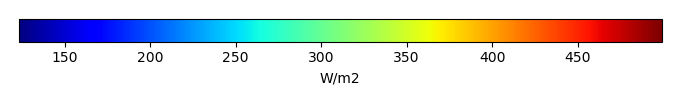MODEL MEANBIAS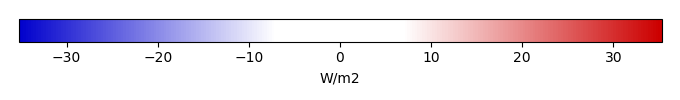BIAS SCORERMSE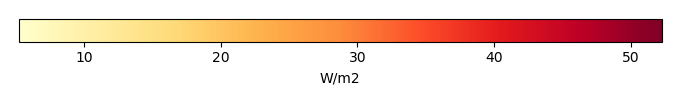RMSE SCOREBENCHMARK INTERANNUAL VARIABILITY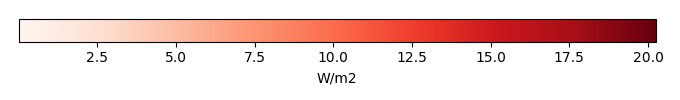MODEL INTERANNUAL VARIABILITYINTERANNUAL VARIABILITY SCOREBENCHMARK MAX MONTHMODEL MAX MONTHDIFFERENCE IN MAX MONTH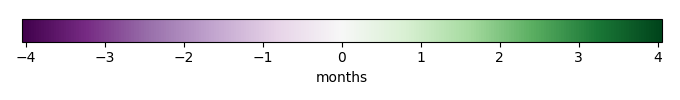SEASONAL CYCLE SCORESPATIAL TAYLOR DIAGRAMMODEL COLORS# Spatially integrated regional mean

MODEL COLORSREGIONAL MEANANNUAL CYCLEMONTHLY ANOMALYANNUAL CYCLE# All Models

BenchmarkCRUNCEPv7GSWP3v1WATCH# Data Information

creation_date: Thu May 8 23:13:11 PDT 2014

source_file: This product is generated from monthly 1 degree CERES EBAF Radiation observations